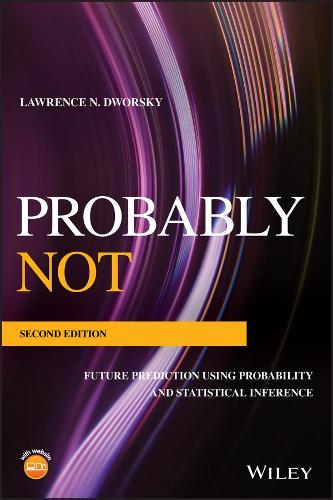•Probably Not: Future Prediction Using Probability and Statistical Inference (Paperback)

(author)
£75.50
Paperback 352 Pages / Published: 04/11/2019
• Coming soon

Awaiting publication

This second edition provides an introduction to probability and statistics at an introductory level, with many timely updates and revisions. The examples provided in the book exemplify how we are living in a statistical world, what we can expect, what we really know based upon the information at hand, and explains when we only think we know something. The book begins with an introduction to probability, followed by an explanation of probability distribution functions. The book then covers building a bell and random walks, with a new section on frequentist interpretation. Combined and conditional probabilities are discussed, with a new section on Bayes Theorem. There is a new chapter on Bayesian Statistics, which features some simple examples, the Presecutor's Paradox, and Bayesian vs. Frequentist thinking about statistics. A new chapter on Benford's Law covers some observations from nature, measuring the compliance, and financial fraud detection using Benford's Law. The book concludes with new material on fractals and mandelbrot sets.

Publisher: John Wiley and Sons Ltd
ISBN: 9781119518105
Number of pages: 352
Weight: 666 g
Edition: 2nd Edition